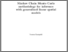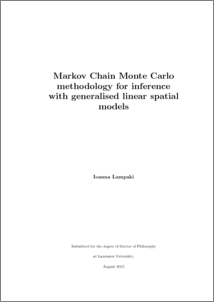# Markov chain Monte Carlo methodoloy for inference with generalised linear spatial models

Lampaki, Ioanna (2016) Markov chain Monte Carlo methodoloy for inference with generalised linear spatial models. PhD thesis, UNSPECIFIED.Preview
PDF (2016LampakiPhD)
2016LampakiPhD.pdf - Published Version

## Abstract

Many real world phenomena are described through models that include an unobserved process which is usually characterised by a continuous distribution. Such models are widely used in geostatistics where a continuous spatial phenomenon is modelled through an underlying latent Gaussian process. If the observed data are also Gaussian then inference for the underlying process and the model parameters is relatively straightforward. In many applications though the assumption of normally distributed data is not sensible and the assumption of Poisson or binomial data is more suitable. These models, with non-Gaussian data, are known as generalised linear spatial models (GLSM). In such cases, inference requires more sophisticated techniques and a common approach is the use of Markov chain Monte Carlo methods (MCMC). However, the correlation between the components of the latent process and the correlation between the latent process and the model parameters generally hinders the performance of any MCMC scheme which updates the latent process and the parameters sequentially. In this thesis we focus on the Poisson GLSM and elaborate on the problem of the correlation within the latent process. In particular, our aim is to construct an efficient proposal distribution for sampling from the posterior distribution of the latent process conditionally on the other parameters. Initially, we investigate the idea of constructing a global normal approximation to the conditional posterior distribution of the latent process and use it as the proposal distribution in a simple and fast MCMC scheme. For this purpose, we initially employ various transformations of the data and find that some of the constructed schemes perform well in certain low dimensional scenarios. Subsequently, we construct one dimensional proposals for each component of the latent process through an approximation to each univariate marginal posterior conditional on a few principal components. The suggested MCMC scheme updates each component of the process separately and then proceeds by updating the few important principal components. As suggested by our results, this method has a stable and efficient performance in a variety of scenarios and dimensions.

Item Type:
Thesis (PhD)
Departments:
ID Code:
79225
Deposited By:
Deposited On:
26 Apr 2016 10:02
Refereed?:
No
Published?:
Published